How Do We Model the Sun?

Overview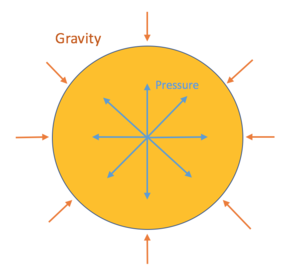Fig. 1: Hydrostatic equilibrium in the sun: pressure pushes outward and gravity pulls inward. (Source: J. Stayner)

How does a star work? It all starts in the core, where atoms are flying around so quickly that they fuse together when they hit each other. The fusion of those atoms produces energy in the form of fast-moving particles that increase the temperature of whatever they bump into. The result of fusion in the core of the star is a self-sustaining explosion of particles at temperatures up to 15 million Kelvin.  The energy from the explosions leaves the core as heat (which spreads toward the outer edges of the sun) and sunlight, which is known scientifically as radiation. This process is described in four main equations that we can use to make a rough model of a star's shape. This treatment of the model draws heavily on Maciel, which derives all the equations in more detail. 

Hydrostatic (Pressure) Equilbrium

To get our first equation, we look at what makes fusion happen in the star. The main interaction here is a battle between gravity and pressure. Gravity pulls inward while pressure pushes outward. A star is formed when the force of gravity is so large that it compresses gases to such temperatures and densities that fusion occurs in its core, where the pressure is the highest. This interaction is also what gives a star its spherical shape: gravity pulls everything toward the core, while pressure pushes outward (as in Fig. 1). Because the radius of a star is fairly constant, we assume that the force due to gravity is equal to the force from pressure, obtaining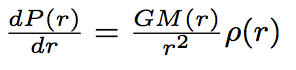(1)

where G is the Newton gravitation constant, M(r) is the total mass contained inside the sphere of radius r, ρ(r) is the mass density at radius r, and P(r) is the pressure at r.

Thermal Equilibrium through Radiation Loss

Our second equation comes from the observation that the sun radiates light but has a fairly constant temperature. Sunlight has quite a bit of energy in it, and if the temperature is constant, then all the energy in sunlight has to be coming from somewhere. Since fusion is the primary source of energy in a star, we relate the luminosity of the star (L) with the rate at which energy is produced per unit mass by nuclear fusion (ε) by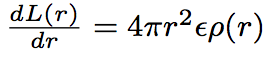(2)

Continuity of Mass

The third equation we will use is based on a way of thinking about the mass of the star; we will use it to find the relationship between the density of the star and its size. The idea is that if the star has a certain density, then we can divide the star into shells with very, very thin thicknesses. Each shell will have a mass dM = 4π r2p(r)dr. We can turn that into a differential equation by dividing by dr, obtaining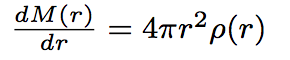(3)

Energy Transport Within the Star

Finally, we know that the sun is hotter at its core than it is on the outside; there are three primary ways this can happen, but only two of them (convection and radiation) are significant to the star's structure.  Radiation in the context of heat flow happens when a part of the star near the core emits light that is then reabsorbed by another part of the star before it can leave. In convection, particles move from one area of the star to another - this is equivalent to a cold wind bringing cooler temperatures to an area. We describe them using the equation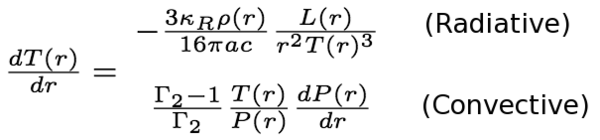(4)

The second expression is used in the convective regions of the star and the radiative expression is used everywhere else. In the Sun, the core is essentially radiative while the outer layers are convective.  Here κR is the radiative opacity, c is the speed of light, T(r) is the temperature in Kelvin, a is Stefan's contant (radiative energy density = aT4), and Γ2 is the dimensionless specific heat ratio of the stellar atmosphere (typically 5/3).

Solving the Equations

We are left with five differential equations, and five obvious variables: M(r), L(r), T(r), P(r), p(r). But, it turns out that ε, Γ2, and κR all depend on the chemical composition of the star as well as some of the other five variables.  As a result, we have six variables with five equations. The solution to that problem is to guess one of the variables - usually the mass M - and then calculate the rest based off of that result. The process is iterated until one comes out with a solution that fits one's observations of the star. In general, this is done with computers due to the tedium of iteration, particularly if you are using the two different energy transport equations for different parts of the star.

© John Stayner. The author grants permission to copy, distribute and display this work in unaltered form, with attribution to the author, for noncommercial purposes only. All other rights, including commercial rights, are reserved to the author.

References

 W. J. Maciel, Introduction to Stellar Structure (Springer, 2016).